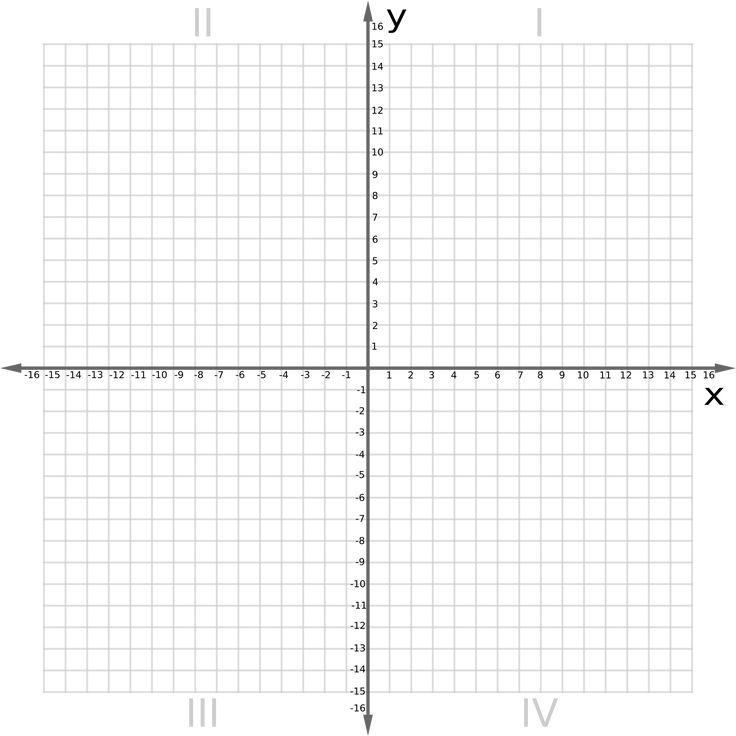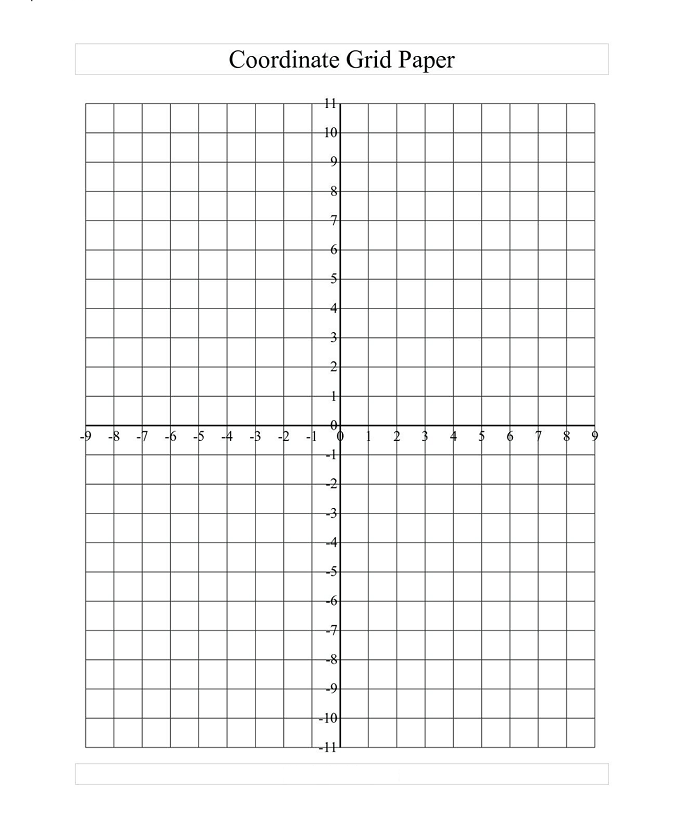# Graph Paper with Axis

Graph paper template with the axis is shared on this page. So if you are in search of graph paper with an axis then simply download it from here and print it out for your use. This type of graph paper is mostly used to plot points on a Cartesian graph. You can see students of mathematics or engineering commonly using these types of graph papers. As you can see in the templates here, there are two lines i.e. X axis and Y axis. Both these lines or axes are drawn perpendicular to each other on graph paper and they intersect at the point “O”. We call these perpendicular straight lines coordinate axes. Both the lines or axes intersect at a point “O” which is known as the origin.

Contents

## Printable Graph Paper with Axis and Numbers

If you are using graph paper for mathematics then it is essential to have proper axis and numbers on it. Making an axis on graph paper by yourself can lead to errors or mistakes, this is why here we have shared these pre-made printable graph paper with axis templates. All you need to do is download a template from here, print it out, and start using it.## Virtual Graph Paper with Axis

• Every endpoint of a square on the x-axis, as illustrated in the figure, indicates a positive integer on the right-hand side of the y-axis.
• Every endpoint of a square on the x-axis, as illustrated in the figure, indicates a negative integer on the left side of the y-axis.
• As seen in the illustration, every y-axis endpoint of a square above the x-axis denotes a positive integer.
• Each end-point of a square on the y-axis, as illustrated in the figure, denotes a negative integer below the x-axis.

### Blank Graph Paper With Axis For Maths

A graph paper with an axis is commonly used for plotting X and Y coordinates to get a visual representation of data. Please note that graph paper is one of the foundational tools of math, science, and art. You may commonly see students of maths and science using these graph papers to plot data, draw objects, etc.